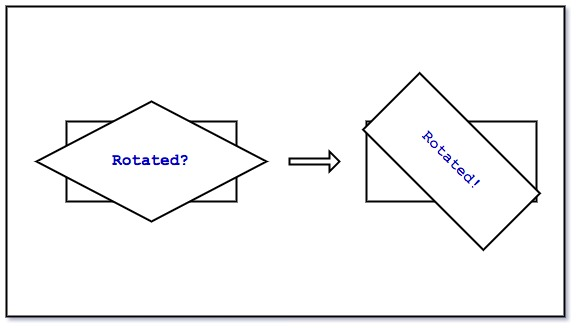To map a texture to a rectangular viewport, we need to define at least 4 vertices for the rectangle, ensure that the texture coordinates are perfectly mapped to the vertices, and call `glDrawArrays(GL_TRIANGLE_STRIP, 0, 4)` to draw the rectangle, this is easy and it works.

Now I want to rotate the texture, say by 45 degrees, this sounds easy too. Simply multiply the vertices by a 4x4 matrix, with the matrix specified with a rotation of 45 degrees or M_PI/4 radians about the Z axis. We can use the OpenGL Math Library or GLM to help create the required matrix:

``````glm::mat4 transform;
transform = glm::rotate(transform, glm::radians(45), glm::vec3(0.0f, 1.0f, 0.0));
``````

This works, but only to some extent, because now the rectangle becomes a rhombus, depending on the degrees rotated, it may become a parallelogram, and the mapped texture is stretched. This is the issue that I am trying to solve in this article.

To make the requirement clearer, I want the mapped texture rotated and its aspect ratio kept, like rotating a monitor(hopefully it's rectangular) on the table, its shape wouldn't change.

Alright, let's get back to the topic. The reason why the mapped texture is stretched and becomes a rhombus is that the transformation is applied on the vertices, which are in NDC (Normalized Device Coordinates) unit, i.e. values ranging from -1 to 1 despite the physical dimensions of the rectangle. Also we have applied on the vertices a linear transformation(a rotation), which has two properties: Lines (or the sides of the rhombus) remain paralell and evenly spaced after being transformed. So either the width or the height of the rectangle will be stretched at this point.

Astute readers will notice that this is an issue related to aspect ratio of the rectangle, because if it is a square, after being rotated by any degrees, it remains a square. So the solution is to take into account the aspect ratio and scale the rectangle itself(or more precisely the vertices that connect to form the rectangle). The next step is to scale the Y axis to respect the aspect ratio:

``````float aspect_ratio = viewport_height / viewport_width;
glm::mat4 transform;
transform = glm::rotate(transform, glm::radians(45), glm::vec3(0.0f, 1.0f, 0.0));
transform = glm::scale(transform, glm::vec3(1.0f, aspect_ratio, 1.0f));
``````

That we scale the Y axis and not the X axis is because we choose height over width and not width over height as the aspect ratio. With the scale, now the rhombus becomes a parallelogram and the mapped texture is squashed along the Y axis (not stretched this time), the reason is that the rotated & scaled vertices in the current coordinate space (Clipped Coordinate space) are entirely mapped to the viewport or the Screen Coordinate space, so squashed rectangle remains squashed rectangle.

To fix the final issue, we will use the aspect ratio again. The idea is to map only a part of the entire Clipped Coordinate space to the viewport, by "a part of ...", you guess right, we use the aspect ratio here. To achieve that, we need to use an orthographic projection matrix:

``````float aspect_ratio = viewport_height / viewport_width;
glm::mat4 transform;
transform = glm::rotate(transform, glm::radians(45), glm::vec3(0.0f, 1.0f, 0.0));
transform = glm::scale(transform, glm::vec3(1.0f, aspect_ratio, 1.0f));
glm::mat4 ortho_proj = glm::ortho(
-1.0f,              // left
1.0f,               // right
-aspect_ratio,      // bottom
aspect_ratio,       // top
0.1f,               // near plane
0.0f                // far plane
) * transform;
``````

The first and second parameter is the left and right of the coordinate space, -1 for left and 1 for right means the entire space along the X axis will be mapped to the X axis of the viewport, the third and forth parameter is the bottom and top of the coordinate space, and we use 'aspect_ratio' as the scalar to select a part of the Clipped Coordinate space along the Y axis to be mapped to the Y axis of the viewport, since orthographic projection is also a linear transformation, this effectively scales the selected part of the Clipped Coordinate space (now aspect ratio aware) to match the viewport (the final Screen Coordinate space), which stretches the parallelogram back to a rectangle.

That's it, the mapped texture in a rectangular viewport is rotated and its aspect ratio is kept.